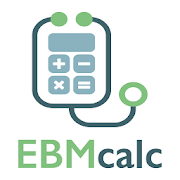# EBMcalc Statistics 16.4.0 APK   
4.3/5
3 Ratings
Developer
Current Version
16.4.0
Date Published
File Size
562k
Package ID
com.medcalc3000.ebmstats
Price
3.99
100+
Category
Android Apps
Genre
Medical

## APK Version History

Version
16.4.0 (Updated)
Architecture
All
Release Date
December 30, 2016
Requirement
Android 4.1 and up
Safe
••••••EBMcalc Statistics

MedCalc 3000 is now EBMcalc!

EBMcalc is the most popular and comprehensive Medical Calculator system on the web. It has been highly acclaimed, reviewed and tested over the last 18 years.

EBMcalc Statistics is your iPhone answer for evidence-based medical calculations and medical statistics. Here you will find essential EBM equations, statistics tools and a a full suite of dose and unit converters ready for use, right in the palm of your hand!

EBMcalc makes the math of EBM simple!

Equations included:

Bayesian Statistics I MultiCalc
Bayesian Statistics II MultiCalc
Benefit Increase / Number Needed to Treat Multicalc
Chi Square Analysis
Confidence Interval of a Binomial Proportion
Confidence Interval of a Difference Between Proportions
Confidence Interval of a Ratio of Proportions
Confidence Interval of a Ratio of Ratios
Confidence Interval of a Survey
COPE Statistic Adding Cost to Number Needed to Treat
False Negative Rate from Sensitivity and Prevalence
False Negative Ratio from Sensitivity and Prevalence
False Positive Rate from Specificity and Prevalence
False Positive Ratio from Specificity and Prevalence
Kappa Measurement of Inter-observer Agreement MultiCalc
Kappa Measurement of Inter-observer Agreement: Bennett's Kappa
Kappa Measurement of Inter-observer Agreement: Cohen's Kappa
Kappa Measurement of Inter-observer Agreement: Scott's Kappa
Likelihood Ratio MultiCalc
Likelihood Ratio of Negative from Raw Data
Likelihood Ratio of Positive from Raw Data
Negative Predictive Value from Raw Data
Negative Predictive Value of a Test
Number Needed to Harm (NNH) from Odds Ratio (OR) and Patient Expected Event Rate (PEER)
Number Needed to Treat (NNT) from Odds Ratio (OR) and Patient Expected Event Rate (PEER)
Odds from Probability
Odds Ratio and Relative Risk
Overall Accuracy of a Test from Raw Data
Overall Accuracy of a Test from Sensitivity, Specificity and Prevalence
Positive Predictive Value from Raw Data
Positive Predictive Value of a Test
Post Test Odds from Pre Test Odds and Likelihood Ratio MultiCalc
Post Test Odds of a Negative from Raw Data
Post Test Odds of a Negative Result from Sensitivity Specificity and Prevalence
Post Test Odds of a Positive from Raw Data
Post Test Odds of a Positive Result from Sensitivity Specificity and Prevalence
Post Test Probability from Pre Test Probability, Sensitivity and Specificity
Post-Test Probability from Likelihood Ratios and Multiple Test Results
Pre Test Odds from Prevalence
Pre Test Odds from Raw Data
Prevalence of a Finding or Disease from Test Data
Probability from Odds
Risk Increase / Number Needed to Harm Multicalc
Risk Reduction / Number Needed to Treat Multicalc
Sensitivity of a Test
Specificity of a Test
Survey Sample Size with Population Correction
Survey Sample Size
True Negative Ratio from Specificity and Prevalence
True Positive Ratio from Sensitivity and Prevalence
Z Score to Percentile Estimation

Some Unit and Dose converters include:

Basic Units
Corticosteroid Dosing
Energy Unit Conversions
Flow Unit Conversions
Pressure Units
SI Unit Conversions
Standard Drink Equivalents
Temperature Unit Conversions
Thyroid Medication Dosing
Time Units
Volume Units
Weight Units

To practice, teach and learn medicine based on solid, literature based evidence, EBMcalc provides easy to use, interactive tools for your Evidence-Based Medicine.

### What's New in this version

New calculators, new favorites functionality, improved navigation.